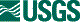## ISIS 2ISIS Application Documentation

# camcoeffs

Standard View | TOC | Home

This program calculates TRANSX,TRANSY from TRANSS, TRANSL for iak kernels

## Description

This application is for camera development and is not supported for general public use. This program outputs the exact IAK Kernel keywords that should be added to the kernel. The TRANSX,TRANSY keywords are the result of the input (no processing done, this should be known information). These values were provides as parameters to this program. The TRANSS,TRANSL keywords are calculated based on the TRANSX,TRANSY keywords and are the result of this program. The keywords can be copied directly into the pertinent IAK kernel.

## Parameter Groups

### IAK

Name Description
IAKCODE The IAK code of the TRANS values

### Coefficients

Name Description
XCONSTCOEF Focal Plane X Constant Coefficient
XSAMPLECOEF Focal Plane X Sample Coefficient
XLINECOEF Focal Plane X Line Coefficient
YCONSTCOEF Focal Plane Y Constant Coefficient
YSAMPLECOEF Focal Plane Y Sample Coefficient
YLINECOEF Focal Plane Y Line Coefficient

### IAK: IAKCODE

#### Description

This IAK code of the TRANS values, a negative number (such as -41210) is expected.

 Type string Output Equations

### Coefficients: XCONSTCOEF

#### Description

This is the first value in the TRANSY keyword, which represents the focal plane X constant coefficient.

 Type double

### Coefficients: XSAMPLECOEF

#### Description

This is the second value in the TRANSY keyword, which represents the focal plane X sample coefficient.

 Type double

### Coefficients: XLINECOEF

#### Description

This is the third value in the TRANSY keyword, which represents the focal plane X line coefficient.

 Type double

### Coefficients: YCONSTCOEF

#### Description

This is the first value in the TRANSY keyword, which represents the focal plane Y constant coefficient.

 Type double

### Coefficients: YSAMPLECOEF

#### Description

This is the second value in the TRANSY keyword, which represents the focal plane Y sample coefficient.

 Type double

### Coefficients: YLINECOEF

#### Description

This is the third value in the TRANSY keyword, which represents the focal plane Y line coefficient.

 Type double

## Examples

### Example 1

Getting the TRANSX,TRANSY keywords for an IAK Kernel

#### Description

This example shows how to get the TRANSX,TRANSY keywords for an IAK Kernel when given the TRANSS, TRANSL keywords

#### Command Line

camcoeffs iakcode=-41219 xc=0.2589180702 xs=-0.0069999992 xl=0.0000033598 yc=-59.9867757282 ys=0.0000033598 yl=-0.0069999992
This is a typical run

#### Data File

The Output This program outputs the exact IAK Kernel keywords that should be added to the kernel. The TRANSX,TRANSY keywords are the result of the input (no processing done, this should be known information). These values were provides as parameters to this program. The TRANSS,TRANSL keywords are calculated based on the TRANSX,TRANSY keywords and are the result of this program. The keywords can be copied directly into the pertinent IAK kernel.

## History

 Steven Lambright 2008-07-16 Original Version